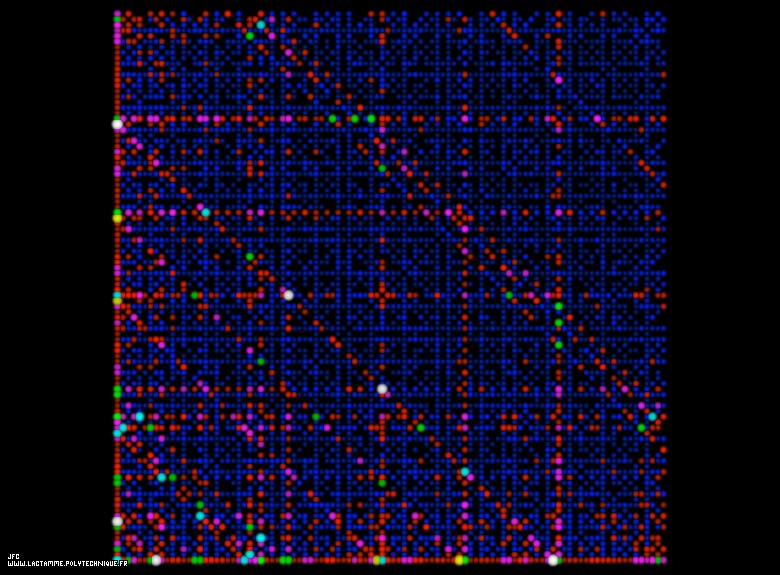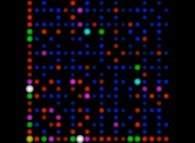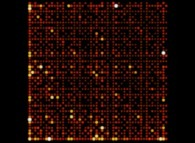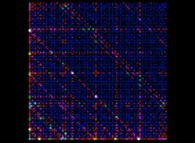The ABC conjecture [La conjecture ABC].

The horizontal and vertical axes display respectively the whole numbers from 1 to N. Each disk display a couple of coprime numbers A (X axis) and B (Y axis):
```                    GCD(A,B)=1
```
The number C is the sum of A and B:
```                    C = A+B
```
The function Radical(N) gives the product of the prime factors (with an exponent equals to 1) of N. For example:
```                                3  1  2
N = 1960 = 2 .5 .7
```
```                                     1  1  1
Radical(1960) = 2 .5 .7  = 2.5.7 = 70
```

Then the following function is computed:
```                                      log(C)
k(A,B,C) = ---------------------
```
The ABC conjecture states that k(A,B,C) is less than a certain constant (unknown, but greater than 1 and hopefully lesser than 2...) whatever the values of A and B.

The surface and the luminance of each disk are proportional to k(A,B,C).

For this picture, the numbers A and B belong to [1,N=100] giving birth to the following values:
```                    min(k(A,B,C))=0.37117806024788
max(k(A,B,C))=1.22629438553090
```

See some related pictures (including this one):(CMAP28 WWW site: this page was created on 09/21/2012 and last updated on 10/05/2015 15:12:44 -CEST-)

[See all related pictures (including this one) [Voir toutes les images associées (incluant celle-ci)]]

[Please visit the related NumberTheory picture gallery [Visitez la galerie d'images NumberTheory associée]]
[Go back to AVirtualMachineForExploringSpaceTimeAndBeyond [Retour à AVirtualMachineForExploringSpaceTimeAndBeyond]]
[The Y2K bug [Le bug de l'an 2000]]

[Site Map, Help and Search [Plan du Site, Aide et Recherche]]
[Mail [Courrier]]
[About Pictures and Animations [A Propos des Images et des Animations]]